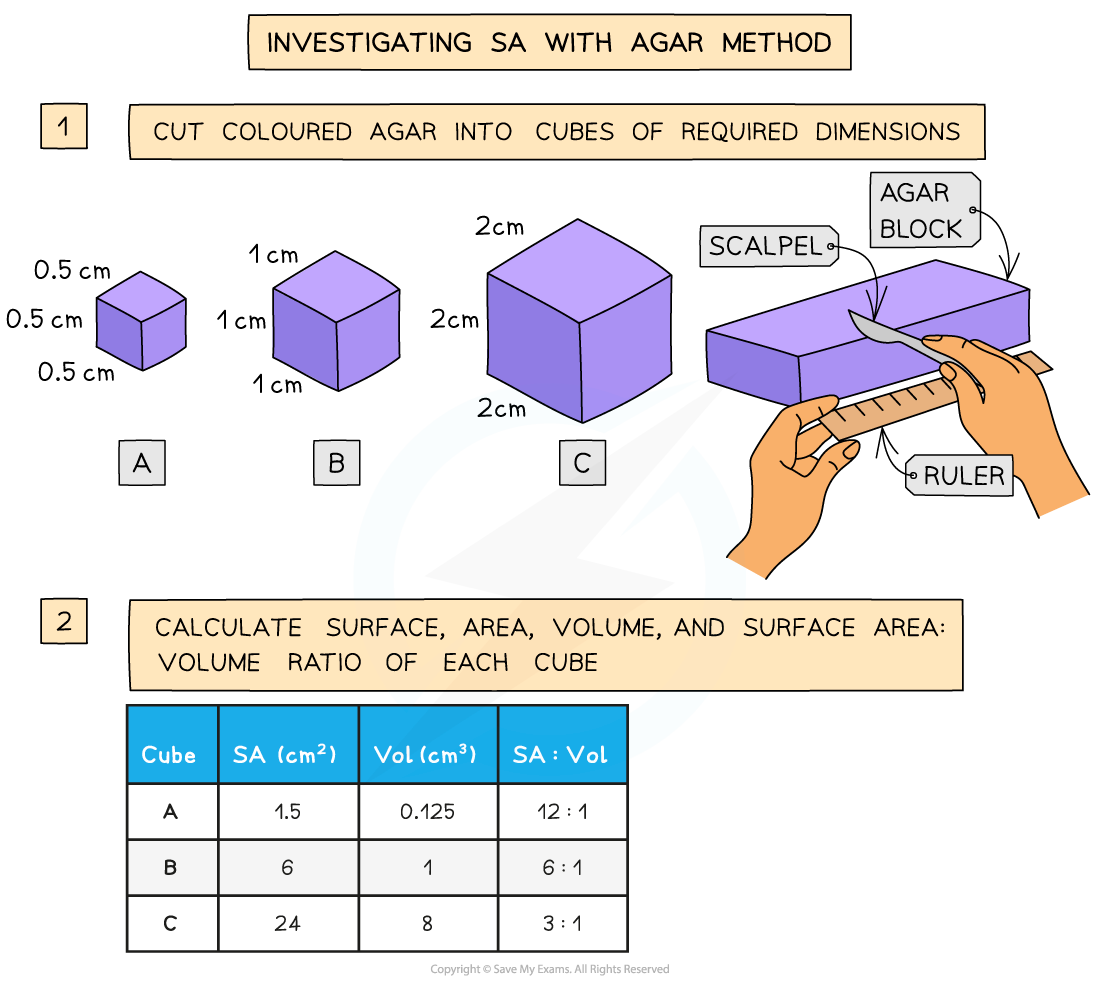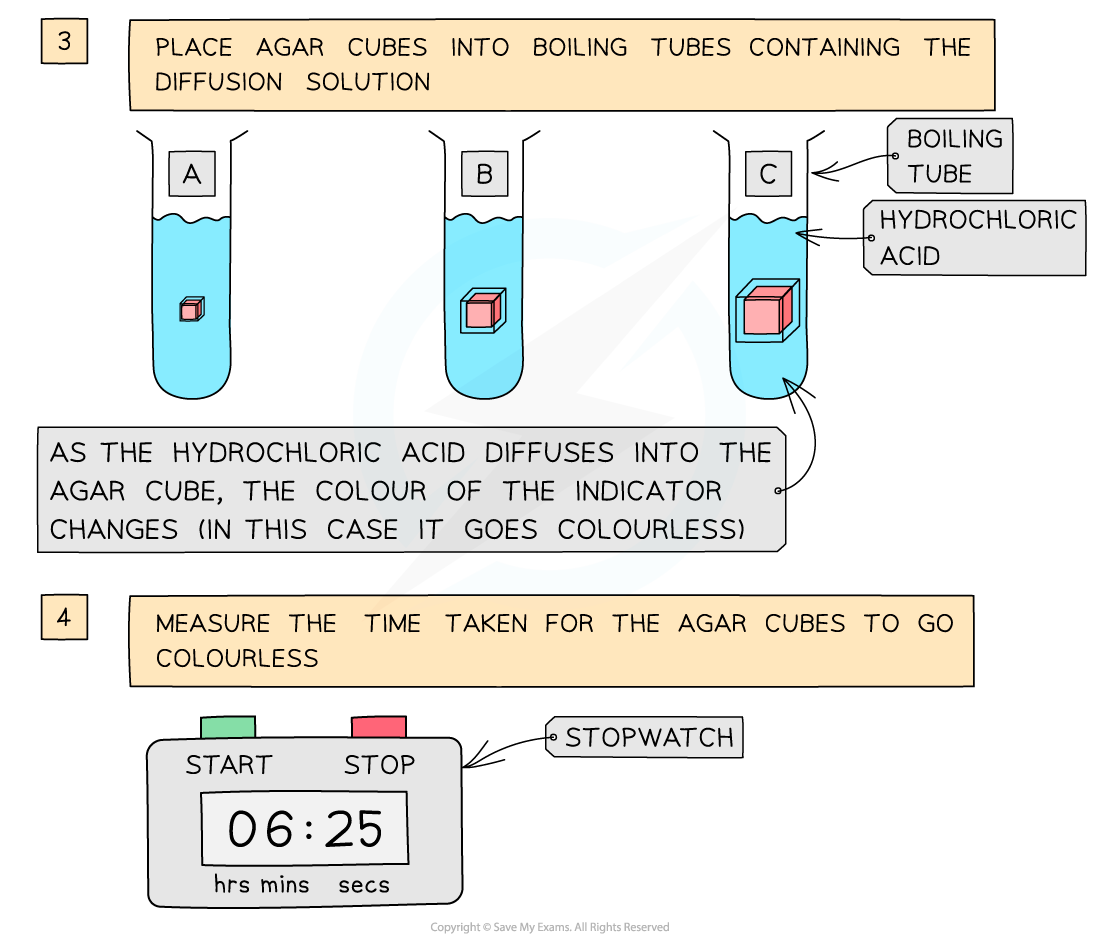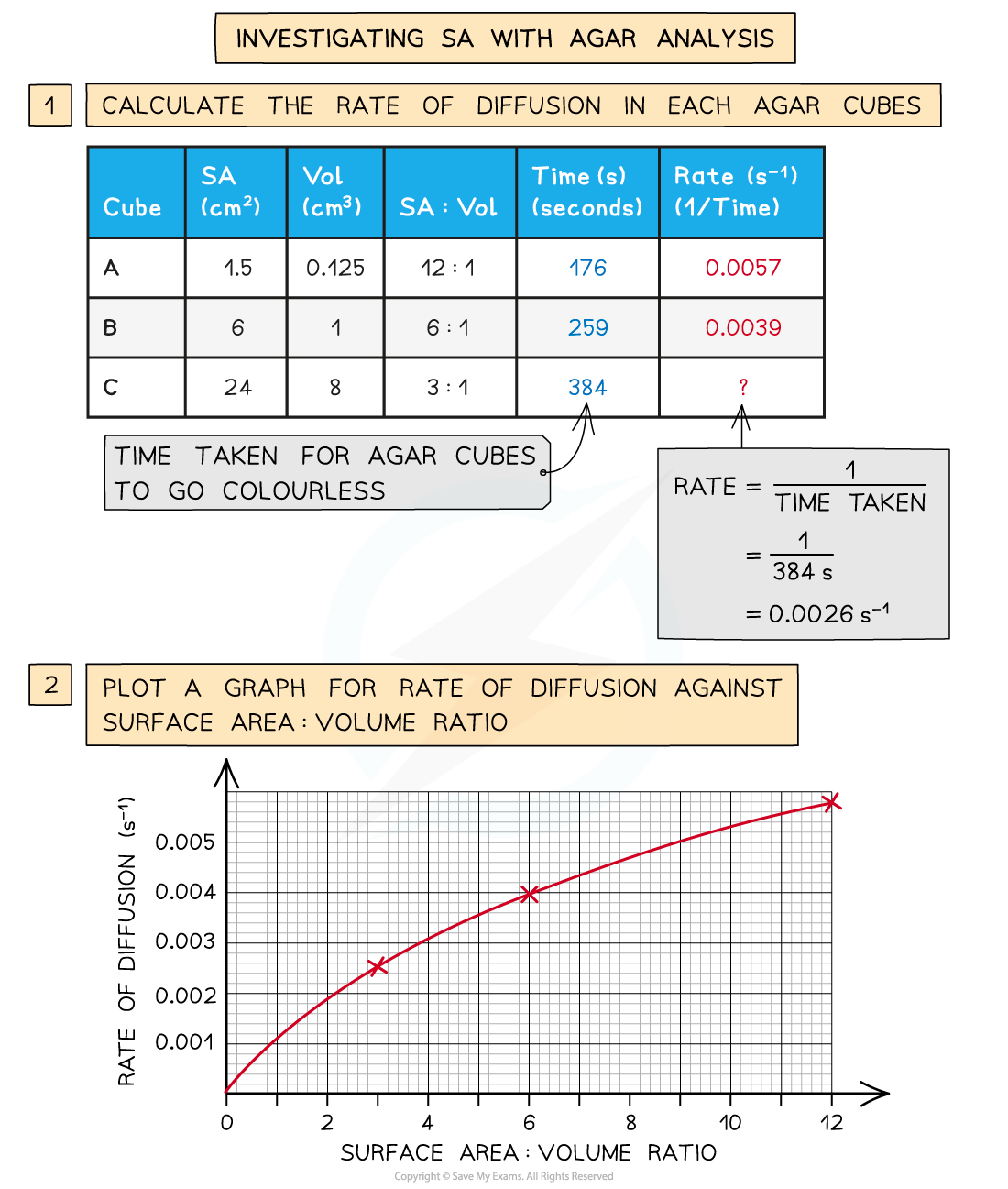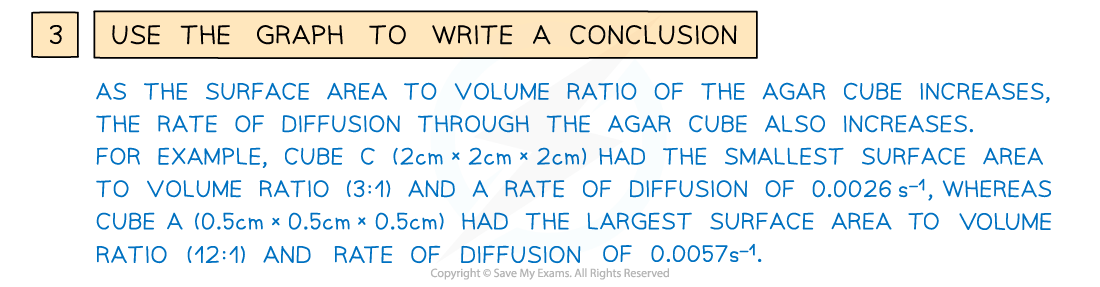# AQA A Level Biology复习笔记3.1.2 Agar Blocks Practical

### Practical Skill: Agar Blocks

• The effect of changing surface area to volume ratio on diffusion can be investigated by timing the diffusion of ions through cubes of agar of different sizes

#### Method

• Coloured agar is made up and cut into cubes of the required dimensions (eg. 0.5cm x 0.5cm x 0.5cm, 1cm x 1cm x 1cm and 2cm x 2cm x 2cm)
• Purple agar can be created if it is made up with very dilute sodium hydroxide solution and Universal Indicator
• Alternatively, the agar can be made up with Universal Indicator only

• The surface area, volume and surface area to volume ratio of these cubes is calculated and recorded
• The cubes are then placed into boiling tubes containing a diffusion solution (such as dilute hydrochloric acid)
• The same volume of dilute hydrochloric acid should be carefully measured out into each boiling tube
• The acid should have higher molarity than the sodium hydroxide so that its diffusion can be monitored by a change in colour of the indicator in the agar blocks

• Measurements can be taken of either:
• The time taken for the acid to completely change the colour of the indicator in the agar blocks
• The distance travelled into the block by the acid (shown by the change in colour of the indicator) in a given time (eg. 5 minutes)The steps used to investigate the effect of changing the surface area to volume ratio on diffusion

#### Analysis

• If the time taken for the acid to completely change the colour of the indicator in the agar blocks is recorded, these times can be converted to rates
• A graph could be drawn showing how the rate of diffusion (rate of colour change) changes with the surface area : volume ratio of the agar cubesTo analyse the results of the investigation, calculate the rates of diffusion before drawing a graph for rate of diffusion against surface area : volume ratio

#### Exam Tip

When an agar cube (or for example a biological cell or organism) increases in size, the volume increases faster than the surface area, because the volume is cubed whereas the surface area is squared. When an agar cube (or biological cell/organism) has more volume but proportionately less surface area, diffusion takes longer and is less effective. In more precise scientific terms, the greater the surface area to volume ratio, the faster the rate of diffusion!